﻿ Essential background II

# Essential background II

Before we tackle the multivariate Kalman Filter, we'll need to review some essential math topics:

• Matrix operations
• Expectation algebra
• Multivariate Normal Distribution

You can jump to the next chapter if you are familiar with these topics.

The notation used in this tutorial:

• Bold-face, lower-case letters refer to vectors, such as $$\boldsymbol{x}$$
• Bold-face, capital letters refer to matrices, such as $$\boldsymbol{A}$$
• Normal-face lower-case letters refer to scalars or vector elements
• Normal-face capital letters refer to matrix elements

## Matrix operations

All you need to know is basic terms and operations such as:

• Vector and matrix addition and multiplication
• Matrix Transpose
• Matrix Inverse (you don't need to invert matrices by yourself, you just need to know what the inverse of the matrix is)
• Symmetric Matrix
• Eigenvalues and eigenvectors

There are numerous Linear Algebra textbooks and web tutorials that cover these topics.

## Expectation algebra

I extensively use the expectation algebra rules for Kalman Filter equations derivations. If you are interested in understanding the derivations, you need to master expectation algebra.

You already know what a random variable is and what an expected value (or expectation) is. If not, please read the Essential background I section.

### Basic expectation rules

The expectation is denoted by the capital letter $$E$$.

The expectation of the random variable $$E(X)$$ equals the mean of the random variable:

$E(X) = \mu_{X}$
Where $$\mu_{X}$$ is the mean of the random variable.

Here are some basic expectation rules:

Rule Notes
1 $$E(X) = \mu_{X} = \Sigma xp(x)$$ $$p(x)$$ is the probability of $$x$$ (discrete case)
2 $$E(a) = a$$ $$a$$ is constant
3 $$E(aX) = aE(X)$$ $$a$$ is constant
4 $$E(a \pm X) = a \pm E(X)$$ $$a$$ is constant
5 $$E(a \pm bX) = a \pm bE(X)$$ $$a$$ and $$b$$ are constant
6 $$E(X \pm Y) = E(X) \pm E(Y)$$ $$Y$$ is another random variable
7 $$E(XY) = E(X)E(Y)$$ If $$X$$ and $$Y$$ are independent

### Variance and Covariance expectation rules

The following table includes the variance and covariance expectation rules.

Rule Notes
8 $$V(a) = 0$$ $$V(a)$$ is the variance of $$a$$
$$a$$ is constant
9 $$V(a \pm X) = V(X)$$ $$V(X)$$ is the variance of $$X$$
$$a$$ is constant
10 $$V(X) = E(X^{2}) - \mu_{X}^{2}$$ $$V(X)$$ is the variance of $$X$$
11 $$COV(X,Y) = E(XY) - \mu_{X}\mu_{Y}$$ $$COV(X,Y)$$ is a covariance of $$X$$ and $$Y$$
12 $$COV(X,Y) = 0$$ if $$X$$ and $$Y$$ are independent
13 $$V(aX) = a^{2}V(X)$$ $$a$$ is constant
14 $$V(X \pm Y) = V(X) + V(Y) \pm 2COV(X,Y)$$
15 $$V(XY) \neq V(X)V(Y)$$

The variance and covariance expectation rules are not straightforward. I prove some of them.

#### Rule 8

$V(a) = 0$

A constant does not vary, so the variance of a constant is 0.

#### Rule 9

$V(a \pm X) = V(X)$

Adding a constant to the variable does not change its variance.

#### Rule 10

$V(X) = E(X^{2}) - \mu_{X}^{2}$

The proof:

Notes
$$V(X) = \sigma_{X}^2 = E((X - \mu_{X})^2)$$
$$= E(X^2 -2X\mu_{X} + \mu_{X}^2)$$
$$= E(X^2) - E(2X\mu_{X}) + E(\mu_{X}^2)$$ Applied rule number 5: $$E(a \pm bX) = a \pm bE(X)$$
$$= E(X^2) - 2\mu_{X}E(X) + E(\mu_{X}^2)$$ Applied rule number 3: $$E(aX) = aE(X)$$
$$= E(X^2) - 2\mu_{X}E(X) + \mu_{X}^2$$ Applied rule number 2: $$E(a) = a$$
$$= E(X^2) - 2\mu_{X}\mu_{X} + \mu_{X}^2$$ Applied rule number 1: $$E(X) = \mu_{X}$$
$$= E(X^2) - \mu_{X}^2$$

#### Rule 11

$COV(X,Y) = E(XY) - \mu_{X}\mu_{Y}$

The proof:

Notes
$$COV(X,Y) = E((X - \mu_{X})(Y - \mu_{Y})$$
$$= E(XY - X \mu_{Y} - Y \mu_{X} + \mu_{X}\mu_{Y})$$
$$= E(XY) - E(X \mu_{Y}) - E(Y \mu_{X}) + E(\mu_{X}\mu_{Y})$$ Applied rule number 6: $$E(X \pm Y) = E(X) \pm E(Y)$$
$$= E(XY) - \mu_{Y} E(X) - \mu_{X} E(Y) + E(\mu_{X}\mu_{Y})$$ Applied rule number 3: $$E(aX) = aE(X)$$
$$= E(XY) - \mu_{Y} E(X) - \mu_{X} E(Y) + \mu_{X}\mu_{Y}$$ Applied rule number 2: $$E(a) = a$$
$$= E(XY) - \mu_{Y} \mu_{X} - \mu_{X} \mu_{Y} + \mu_{X}\mu_{Y}$$ Applied rule number 1: $$E(X) = \mu_{X}$$
$$= E(XY) - \mu_{X}\mu_{Y}$$

#### Rule 13

$V(aX) = a^{2}V(X)$

The proof:

Notes
$$V(K) = \sigma_{K}^2 = E(K^{2}) - \mu_{K}^2$$
$$K = aX$$
$$V(K) = V(aX) = E((aX)^{2} ) - (a \mu_{X})^{2}$$ Substitute $$K$$ with $$aX$$
$$= E(a^{2}X^{2}) - a^{2} \mu_{X}^{2}$$
$$= a^{2}E(X^{2}) - a^{2}\mu_{X}^{2}$$ Applied rule number 3: $$E(aX) = aE(X)$$
$$= a^{2}(E(X^{2}) - \mu_{X}^{2})$$
$$= a^{2}V(X)$$ Applied rule number 10: $$V(X) = E(X^{2}) - \mu_{X}^2$$

For constant velocity motion:

$V(x) = \Delta t^{2}V(v)$ or $\sigma_{x}^2 = \Delta t^{2}\sigma_{v}^2$
Where:
 $$x$$ is the displacement of the body $$v$$ is the velocity of the body $$\Delta t$$ is the time interval

#### Rule 14

$V(X \pm Y) = V(X) + V(Y) \pm 2COV(X,Y)$

The proof:

Notes
$$V(X \pm Y)$$
$$= E((X \pm Y)^{2}) - (\mu_{X} \pm \mu_{Y})^{2}$$ Applied rule number 10: $$V(X) = E(X^{2}) - \mu_{X}^2$$
$$= E(X^{2} \pm 2XY + Y^{2}) - (\mu_{X}^2 \pm 2\mu_{X}\mu_{Y} + \mu_{y}^2)$$
$$= \color{red}{E(X^{2}) - \mu_{X}^2} + \color{blue}{E(Y^{2}) - \mu_{Y}^2} \pm 2(E(XY) - \mu_{X}\mu_{Y} )$$ Applied rule number 6: $$E(X \pm Y) = E(X) \pm E(Y)$$
$$= \color{red}{V(X)} + \color{blue}{V(Y)} \pm 2(E(XY) - \mu_{X}\mu_{Y} )$$ Applied rule number 10: $$V(X) = E(X^{2}) - \mu_{X}^2$$
$$= V(X) + V(Y) \pm 2COV(X,Y)$$ Applied rule number 11: $$COV(X,Y) = E(XY) - \mu_{X}\mu_{Y}$$

## Multivariate Normal Distribution

### Introduction

We've seen that the Kalman Filter output is a random variable. The mean of the random variable represents the state estimate. The variance of the random variable is the estimation uncertainty. The Kalman Filter provides us with the estimate and the level of confidence of its estimate.

The one-dimensional Kalman Filter equations include four uncertainty variables:

• $$p_{n,n}$$ is the variance of an estimate (the current state)
• $$p_{n+1,n}$$ is the variance of a prediction (the next state)
• $$r_{n}$$ is the measurement variance
• $$q$$ is the process noise

For a multivariate Kalman Filter, the system state is described by a vector with more than one variable. For example, the object's position on the plane can be described by two variables: x – position and y – position:

$\boldsymbol{x} = \left[ \begin{matrix} x\\ y\\ \end{matrix} \right]$

The Kalman Filter output is a multivariate random variable. A covariance matrix describes the squared uncertainty of the multivariate random variable.

The uncertainty variables of the multivariate Kalman Filter are:

• $$\boldsymbol{P}_{n,n}$$ is a covariance matrix that describes the squared uncertainty of an estimate
• $$\boldsymbol{P}_{n+1,n}$$ is a covariance matrix that describes the squared uncertainty of a prediction
• $$\boldsymbol{R}_{n}$$ is a covariance matrix that describes the squared measurement uncertainty
• $$\boldsymbol{Q}$$ is a covariance matrix that describes the process noise

This chapter is about the multivariate normal distribution and the covariance matrix.

### Covariance

Covariance is a measure of the strength of the correlation between two or more sets of random variates.

Assume a set of object location measurements on the $$x-y$$ plane.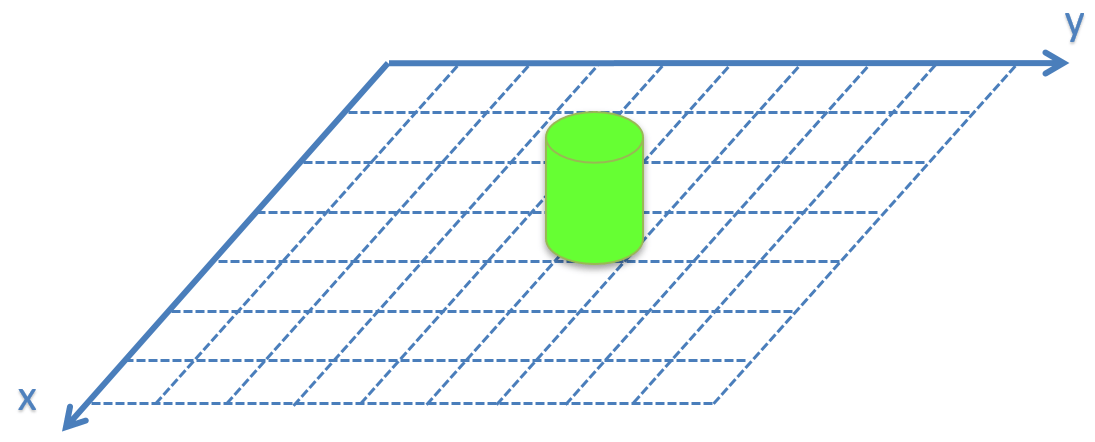Due to the random error, there is a variance in the measurements.

Let us see some examples of different measurement sets.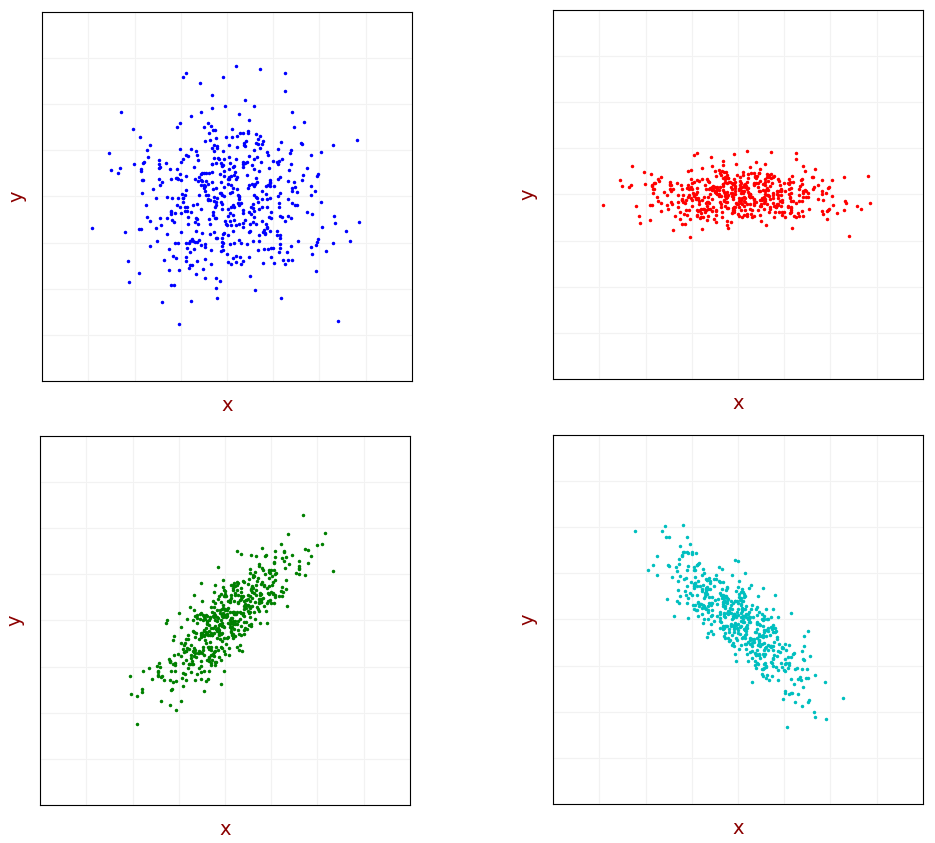The two upper subplots demonstrate uncorrelated measurements. The $$x$$ values don't depend on $$y$$ values. The $$x$$ and $$y$$ values of the blue data set have the same variance, and we can see a circular distribution shape. For the red data set, the variance of the $$x$$ values is greater than that of the $$y$$ values, and the distribution shape is elliptic.

Since the measurements are uncorrelated, the covariance of $$x$$ and $$y$$ equals zero.

The two lower subplots demonstrate correlated measurements. There is a dependency between $$x$$ and $$y$$ values. For the green data set, an increase in $$x$$ results in an increase in $$y$$ and vice versa. The correlation is positive; therefore, the covariance is positive. For the cyan data set, an increase in $$x$$ results in a decrease in $$y$$ and vice versa. The correlation is negative; therefore, the covariance is negative.

The covariance between population $$X$$ and population $$Y$$ with size $$N$$ is given by:

$COV(X,Y) = \frac{1}{N}\sum_{i=1}^{N}(x_{i} - \mu_{x})(y_{i} - \mu_{y})$

Let us rewrite the covariance equation:

Notes
$$COV(X,Y) = \frac{1}{N}\sum_{i=1}^{N}(x_{i} - \mu_{x})(y_{i} - \mu_{y})$$
$$= \frac{1}{N}\sum_{i=1}^{N}(x_{i}y_{i} - x_{i}\mu_{y} - y_{i}\mu_{x} + \mu_{x}\mu_{y})$$ Open parenthesis
$$= \frac{1}{N}\sum_{i=1}^{N}(x_{i}y_{i}) - \frac{1}{N}\sum_{i=1}^{N}(x_{i}\mu_{y}) - \frac{1}{N}\sum_{i=1}^{N}(y_{i}\mu_{x}) + \frac{1}{N}\sum_{i=1}^{N}(\mu_{x}\mu_{y})$$ Distribute
$$= \frac{1}{N}\sum_{i=1}^{N}(x_{i}y_{i}) - \frac{\mu_{y}}{N}\sum_{i=1}^{N}(x_{i}) - \frac{\mu_{x}}{N}\sum_{i=1}^{N}(y_{i}) + \mu_{x}\mu_{y}$$ $$\mu_{x} = \frac{1}{N}\sum_{i=1}^{N}(x_{i}); \mu_{y} = \frac{1}{N}\sum_{i=1}^{N}(y_{i})$$
$$= \frac{1}{N}\sum_{i=1}^{N}(x_{i}y_{i}) - \mu_{x}\mu_{y} - \mu_{x}\mu_{y} + \mu_{x}\mu_{y}$$
$$= \frac{1}{N}\sum_{i=1}^{N}(x_{i}y_{i}) - \mu_{x}\mu_{y}$$

The covariance of a sample with size $$N$$ is normalized by $$N-1$$:

$COV(X,Y) = \frac{1}{N-1}\sum_{i=1}^{N}(x_{i} - \mu_{x})(y_{i} - \mu_{y})$

Let us rewrite the covariance equation:

Notes
$$COV(X,Y) = \frac{1}{N-1}\sum_{i=1}^{N}(x_{i} - \mu_{x})(y_{i} - \mu_{y})$$
$$= \frac{1}{N-1}\sum_{i=1}^{N}(x_{i}y_{i} - x_{i}\mu_{y} - y_{i}\mu_{x} + \mu_{x}\mu_{y})$$ Open parenthesis
$$= \frac{1}{N-1}\sum_{i=1}^{N}(x_{i}y_{i}) - \frac{1}{N-1}\sum_{i=1}^{N}(x_{i}\mu_{y}) - \frac{1}{N-1}\sum_{i=1}^{N}(y_{i}\mu_{x}) + \frac{1}{N-1}\sum_{i=1}^{N}(\mu_{x}\mu_{y})$$ Distribute
$$= \frac{1}{N-1}\sum_{i=1}^{N}(x_{i}y_{i}) - \frac{\mu_{y}}{N-1}\sum_{i=1}^{N}(x_{i}) - \frac{\mu_{x}}{N-1}\sum_{i=1}^{N}(y_{i}) + \frac{N}{N-1}\mu_{x}\mu_{y}$$ $$\mu_{x} = \frac{1}{N}\sum_{i=1}^{N}(x_{i}); \mu_{y} = \frac{1}{N}\sum_{i=1}^{N}(y_{i})$$
$$= \frac{1}{N-1}\sum_{i=1}^{N}(x_{i}y_{i}) - \frac{N}{N-1}\mu_{x}\mu_{y} - \frac{N}{N-1}\mu_{x}\mu_{y} + \frac{N}{N-1}\mu_{x}\mu_{y}$$
$$= \frac{1}{N-1}\sum_{i=1}^{N}(x_{i}y_{i}) - \frac{N}{N-1}\mu_{x}\mu_{y}$$

Example:

Given samples:

$\boldsymbol{x} = \left[ \begin{matrix} 2 \\ 3 \\ -1 \\ 4 \end{matrix} \right]$

$\boldsymbol{y} = \left[ \begin{matrix} 8 \\ 7 \\ 9 \\ 6 \end{matrix} \right]$

$COV(X,Y) = \frac{1}{N-1}\sum_{i=1}^{N}(x_{i}y_{i}) - \frac{N}{N-1}\mu_{x}\mu_{y} =$

$= \frac{1}{3} \left( 2 \times 8 + 3 \times 7 - 1 \times 9 + 4 \times 6 \right) - \frac{4}{3} \left( \frac{(2+3-1+4)}{4} \frac{(8+7+9+6)}{4} \right) = -2.67$

We can stack the samples in two vectors $$\boldsymbol{x}$$ and $$\boldsymbol{y}$$. The covariance in vector notation is given by:

$COV(X,Y) = \frac{1}{N-1}\boldsymbol{x}^{T}\boldsymbol{y} - \frac{N}{N-1}\mu_{x}\mu_{y}$

For a zero-mean random variable, the covariance is given by:

$COV(X,Y) = \frac{1}{N-1}\boldsymbol{x}^{T}\boldsymbol{y}$

### Covariance matrix

A covariance matrix is a square matrix that represents the covariance between each pair of elements in a given multivariate random variable.

For a two-dimensional random variable, the covariance matrix is:

$\boldsymbol{\Sigma} = \left[ \begin{matrix} \sigma_{xx} & \sigma_{xy} \\ \sigma_{yx} & \sigma_{yy} \\ \end{matrix} \right] = \left[ \begin{matrix} \sigma_{x}^{2} & \sigma_{xy} \\ \sigma_{yx} & \sigma_{y}^{2} \\ \end{matrix} \right] = \left[ \begin{matrix} VAR(\boldsymbol{x}) & COV(\boldsymbol{x, y}) \\ COV(\boldsymbol{y, x}) & VAR(\boldsymbol{y}) \\ \end{matrix} \right]$

Note that the off-diagonal entries of the covariance matrix are equal since $$COV(\boldsymbol{x, y}) = COV(\boldsymbol{y, x})$$. If $$x$$ and $$y$$ are uncorrelated, the off-diagonal entries of the covariance matrix are zero.

For a $$n$$ - dimensional random variable, the covariance matrix is:

$\boldsymbol{\Sigma} = \left[ \begin{matrix} \sigma_{1}^{2} & \sigma_{12} & \cdots & \sigma_{1n} \\ \sigma_{21} & \sigma_{2}^{2} & \cdots & \sigma_{2n} \\ \vdots & \vdots & \ddots & \vdots \\ \sigma_{n1} & \sigma_{n2} & \cdots & \sigma_{n}^{2}\\ \end{matrix} \right]$

Most scientific computer packages can compute the covariance matrix.


import numpy as np

x = np.array([2, 3, -1, 4])
y = np.array([8, 7, 9, 6])

C = np.cov(x,y)
print(C)

[[ 4.66666667 -2.66666667]
[-2.66666667  1.66666667]]


x = [2 3 -1 4];
y = [8 7 9 6];

C = cov(x,y)

C =

4.6667   -2.6667
-2.6667    1.6667


#### Properties of the covariance matrix

1. The diagonal entries of this covariance matrix are the variances of the components of the multivariate random variable.
2. $\boldsymbol{\Sigma}_{ii} = \sigma^{2}_{i}$

3. Since the diagonal entries are all non-negative, the trace (the sum of diagonal entries) of this covariance matrix is non-negative:
4. $tr(\boldsymbol{\Sigma}) = \sum^{n}_{i=1}\boldsymbol{\Sigma}_{ii} \geq 0$

5. Since $$\boldsymbol{\Sigma}_{ij} = \sigma_{ij} = \sigma_{ji} = \boldsymbol{\Sigma}_{ji}$$, the covariance matrix is symmetric:
6. $\boldsymbol{\Sigma} = \boldsymbol{\Sigma}^{T}$

7. The covariance matrix is positive semidefinite.
The matrix $$\boldsymbol{A}$$ is called positive semidefinite if, for any vector $$\boldsymbol{v} \neq 0, \boldsymbol{v}^{T}\boldsymbol{A}\boldsymbol{v} \geq 0$$.
The eigenvalues of $$\boldsymbol{A}$$ are non-negative.

#### Covariance matrix and expectation

Assume vector $$\boldsymbol{x}$$ with $$k$$ elements:

$\boldsymbol{x} = \left[ \begin{matrix} x_{1}\\ x_{2}\\ \vdots \\ x_{k}\\ \end{matrix} \right]$

The entries of the vector are random variables with finite variance. The covariance matrix of the vector $$\boldsymbol{x}$$ is given by:

$COV(\boldsymbol{x}) = E \left( \left( \boldsymbol{x} - \boldsymbol{\mu}_{x} \right) \left( \boldsymbol{x} - \boldsymbol{\mu}_{x} \right)^{T} \right)$

The proof:

$COV(\boldsymbol{x}) = E \left( \left[ \begin{matrix} (x_{1} - \mu_{x_{1}})^{2} & (x_{1} - \mu_{x_{1}})(x_{2} - \mu_{x_{2}}) & \cdots & (x_{1} - \mu_{x_{1}})(x_{k} - \mu_{x_{k}}) \\ (x_{2} - \mu_{x_{2}})(x_{1} - \mu_{x_{1}}) & (x_{2} - \mu_{x_{2}})^{2} & \cdots & (x_{2} - \mu_{x_{2}})(x_{k} - \mu_{x_{k}}) \\ \vdots & \vdots & \ddots & \vdots \\ (x_{k} - \mu_{x_{k}})(x_{1} - \mu_{x_{1}}) & (x_{k} - \mu_{x_{k}})(x_{2} - \mu_{x_{2}}) & \cdots & (x_{k} - \mu_{x_{k}})^{2} \\ \end{matrix} \right] \right) =$

$= E \left( \left[ \begin{matrix} (x_{1} - \mu_{x_{1}}) \\ (x_{2} - \mu_{x_{2}}) \\ \vdots \\ (x_{k} - \mu_{x_{k}}) \\ \end{matrix} \right] \left[ \begin{matrix} (x_{1} - \mu_{x_{1}}) & (x_{2} - \mu_{x_{2}}) & \cdots & (x_{k} - \mu_{x_{k}}) \end{matrix} \right] \right) =$

$= E \left( \left( \boldsymbol{x} - \boldsymbol{\mu}_{x} \right) \left( \boldsymbol{x} - \boldsymbol{\mu}_{x} \right)^{T} \right)$

### Multivariate normal distribution

We are already familiar with a univariate normal distribution. It is described by a bell-shaped Gaussian function:

$p(x|\mu,\sigma) = \frac{1}{\sqrt{2\pi\sigma^{2}}} exp \left( -\frac{(x-\mu)^{2}}{2\sigma^{2}} \right)$

The normal distribution is denoted by:

$\mathcal{N}(\mu,\sigma^{2})$

The multivariate normal distribution is a generalization of the univariate normal distribution for a multidimensional random variable.

The $$n$$ - dimensional multivariate normal distribution is described by:

$p(\boldsymbol{x}|\boldsymbol{\mu},\boldsymbol{\Sigma}) = \frac{1}{\sqrt{(2\pi)^{n}|\boldsymbol{\Sigma}|}} exp \left( -\frac{1}{2}(\boldsymbol{x} - \boldsymbol{\mu})^{T}\boldsymbol{\Sigma}^{-1}(\boldsymbol{x} - \boldsymbol{\mu}) \right)$

Where:

• $$\boldsymbol{x}$$ is an $$n$$ - dimensional random vector
• $$\boldsymbol{\mu}$$ is an $$n$$ - dimensional mean vector
• $$\boldsymbol{\Sigma}$$ is a square $$n \times n$$ covariance matrix

### Bivariate normal distribution

A bivariate (two-dimensional) normal distribution comprises two normally distributed random variables. I want to focus on the bivariate normal distribution since it is the highest dimension of the multivariate normal distribution that we can visualize.

The following plot describes the bivariate Gaussian function.### Confidence intervals

A confidence interval specifies the probability that a parameter falls between a pair of values around the mean for the univariate normal distribution.

For the univariate normal distribution, the area between the $$1\sigma$$ boundaries and the Gaussian function is 68.26% of the total area under the Gaussian function.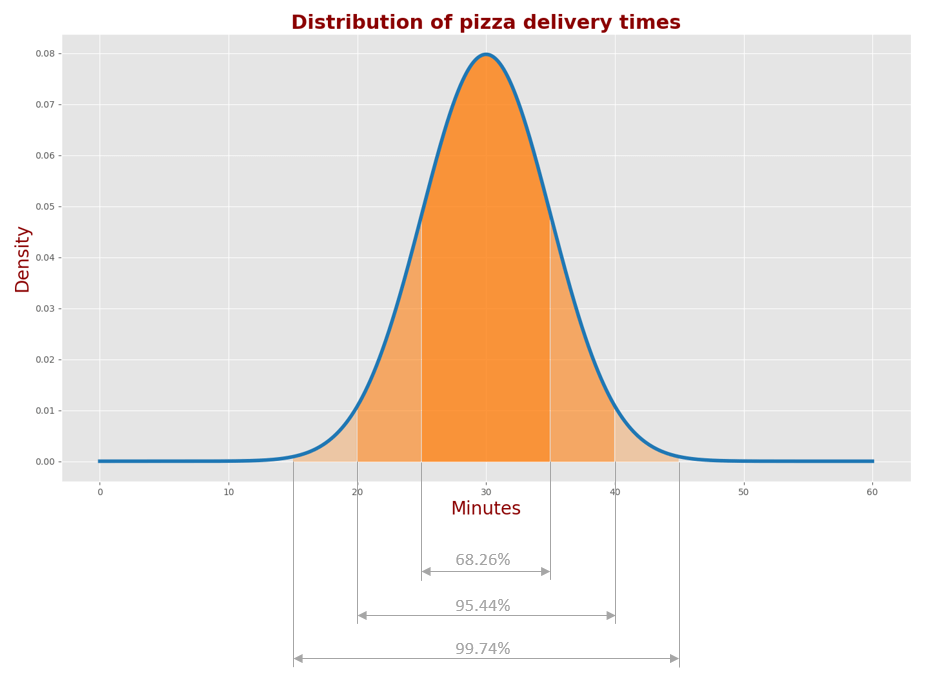For the univariate normal distribution, we can formulate the following statements:

• The confidence interval for the probability of 68.26% is $$1\sigma$$.
• The confidence interval for the probability of 95.44% is $$2\sigma$$.
• The confidence interval for the probability of 99.74% is $$3\sigma$$.

We can also find the confidence interval of any specified probability for the univariate normal distribution. You can see the explanation here.

The probability of the bivariate normal distribution is a volume of the three-dimensional Gaussian function.

For example, the volume of the three-dimensional Gaussian function sliced at $$1\sigma$$ level is 39.35% of the total volume of the three-dimensional Gaussian function.

The projection of the three-dimensional Gaussian slice is an ellipse.### Covariance ellipse

First, let us find the properties of the covariance ellipse. The covariance ellipse represents an iso-contour of the Gaussian distribution and allows visualization of a $$1\sigma$$ confidence interval in two dimensions. The covariance ellipse provides a geometric interpretation of the covariance matrix.

Any ellipse can be described by four parameters:

• Ellipse center $$\mu_{x}, \mu_{y}$$
• Half-major axis $$a$$
• Half-minor $$b$$
• Orientation angle $$\theta$$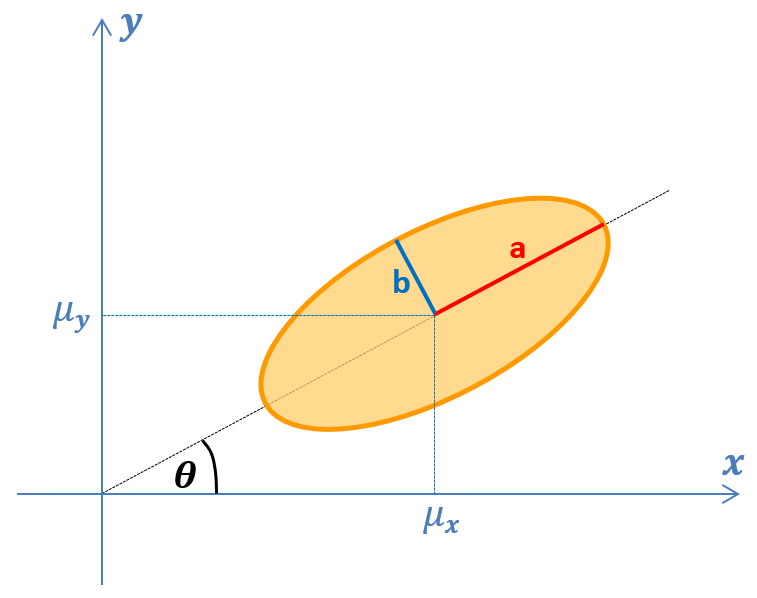The ellipse center is a mean of the random variable:

$\mu_{x} = \frac{1}{N}\sum^{N}_{i=1}x_{i}$

$\mu_{y} = \frac{1}{N}\sum^{N}_{i=1}y_{i}$

The lengths of the ellipse axes are the square roots of the eigenvalues of the random variable covariance matrix:

• The length of the half-major axis $$a$$ is given by the highest eigenvalue square root
• The length of the half-minor axis $$b$$ is given by the second eigenvalue square root

The orientation of the ellipse is an orientation of the covariance matrix eigenvector that corresponds to the highest eigenvalue.

$\theta = arctan \left( \frac{v_{y}}{v_{x}} \right)$

Where:

• $$v_{x}$$ is the $$x$$ - coordinate of the eigenvector that corresponds to the highest eigenvalue
• $$v_{y}$$ is the $$y$$ - coordinate of the eigenvector that corresponds to the highest eigenvalue

You can use a scientific computer package to compute the covariance ellipse parameters.


import numpy as np

C = np.array([[5, -2],[-2, 1]]) # define covariance matrix

eigVal, eigVec = np.linalg.eig(C) # find eigenvalues and eigenvectors

a = np.sqrt(eigVal) # half-major axis length
b = np.sqrt(eigVal) # half-minor axis length

# ellipse  orientation  angle
theta = np.arctan(eigVec[1, 0] / eigVec[0, 0])


C = [5 -2; -2 1]; % define covariance matrix

[eigVec, eigVal] = eig(C); % find eigenvalues and eigenvectors

if eigVal(1,1) > eigVal(2,2) % get the highest eigenvalue index

a = sqrt(eigVal(1,1)); % half-major axis length
b = sqrt(eigVal(2,2)); % half-minor axis length

theta = atan(eigVec(2,1) / eigVec(1,1));  % ellipse angle (radians)
else

a = sqrt(eigVal(2,2)); % half-major axis length
b = sqrt(eigVal(1,1)); % half-minor axis length

theta = atan(eigVec(2,2) / eigVec(2,1));  % ellipse angle (radians)
end


### Confidence ellipse

In many applications, there is an interest in finding the boundaries of specific probability. For example, for 95% probability, we should find the boundary that includes 95% of the Gaussian function volume.

The projection of this boundary onto the $$x-y$$ plane is the confidence ellipse.

We want to find an elliptical scale factor $$k$$, that extends the covariance ellipse to the confidence ellipse associated with 95% probability.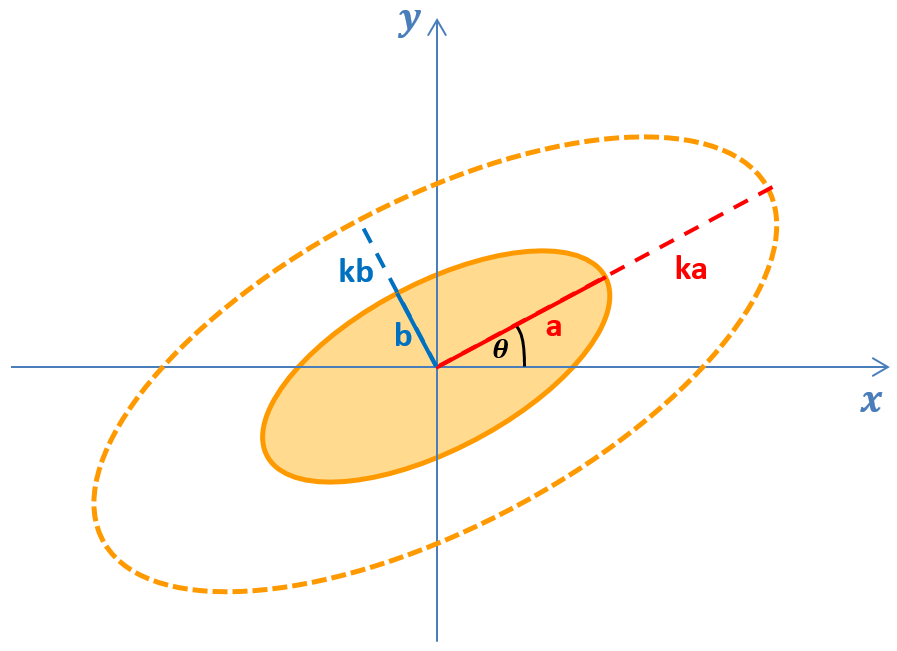Since $$\sigma_{x}$$ and $$\sigma_{y}$$ represent the standard deviations of stochastically independent random variables, the addition theorem for the chi-square distribution may be used to show that the probability associated with a confidence ellipse is given by:

$p = 1 - exp \left( -\frac{1}{2}k^{2} \right)$

For a covariance ellipse $$k = 1$$, therefore, the probability associated with a covariance ellipse is:

$p = 1 - exp \left( -\frac{1}{2} \right) = 39.35\%$

For a given probability, we can find an elliptical scale factor:

$k = \sqrt{-2ln(1-p)}$

For the probability of 95%:

$k = \sqrt{-2ln(1-0.95)} = 2.45$

The properties of the confidence ellipse associated with 95% probability are:

• Ellipse center $$(\mu_{x}, \mu_{y})$$ is similar to the covariance ellipse
• Orientation angle $$\theta$$ is similar to the covariance ellipse
• Half-major axis length is $$2.45a$$ – a scaled half-major axis of the covariance ellipse
• Half-minor axis length is $$2.45b$$ – a scaled half-minor axis of the covariance ellipse